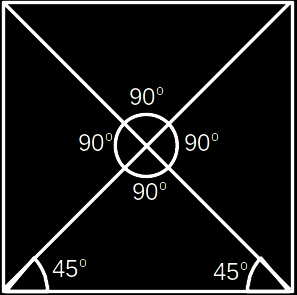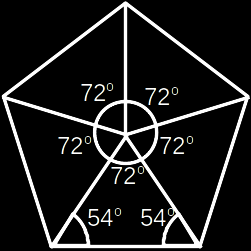# How many degrees does the internal angle of a regular polygon with infinite sides?

Who has never seen himself questioning how many degrees each internal angle of a regular polygon with infinite sides should have? Most popularly called the regular infinitone. This should be a recurring question for most people (at least those with enough time and patience to read this blog).

To analyze this problem, we will start from the fact that the sum of the internal angles of a triangle is 180 degrees. A very simple math, but that we will show to be sufficient for this calculation with a regular infinitone.

In this case, if the regular triangle has all the same angles (by definition of being a regular polygon), then each internal angle of it must be 180/3 degrees. That is, 60 degrees.In the case of a square (a regular 4-sided polygon). We can divide a square from its center and thus create 4 triangles. The sum of the angles of its center should give a total of 360 degrees, as they are complete. Dividing 360 degrees by the number of sides, in case 4, we arrive that the sum of the other two angles must be equal to the internal angle of the polygon, in this case, 45 + 45 degrees, that is, 90 degrees.In the case of a regular pentagon (a regular 5-sided polygon). We can divide it from its center and thus create 5 triangles. The sum of the angles of its center should give a total of 360 degrees, as they are complete. Dividing 360 degrees by the number of sides, in case 5, we arrive that the sum of the other two angles must be equal to the internal angle of the polygon, in this case, 54 + 54 degrees, that is, 108 degrees.For the square, the answer could be intuitive, but the reasoning is used both for the pentagon (as shown above) and for figures from more sides.

6 sides: [180 – (360/6)] = 120 degrees

7 sides: [180 – (360/7)] = 128.5 degrees

8 sides: [180 – (360/8)] = 135 degrees

9 sides: [180 – (360/9)] = 140 degrees

10 sides: [180 – (360/10)] = 144 degrees

100 sides: [180 – (360/100)] = 176,4 degrees

1.000 sides: [180 – (360/1.000)] = 179,6 degrees

1.000.000 sides: [180 – (360/1.000.000)] = 179,9996 degrees

1.000.000.000 sides: [180 – (360/1.000.000.000)] = 179,9999996 degrees

Applying a simple limit to the degrees function of this regular polygon, we can arrive at the following expression:

180 – lim (360/x) = 180 – 0 = 180.
x→∞

That is, when the number of sides tends to infinity, the measure of each internal angle of the polygon is 180 degrees. Incredible to think how it closes only with shallow angles.

### One thought on “How many degrees does the internal angle of a regular polygon with infinite sides?”

• Pingback: URL